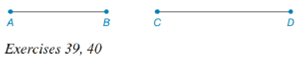Chapter 7.1, Problem 40EElementary Geometry For College St...

7th Edition
Alexander + 2 others
ISBN: 9781337614085

Solutions

Chapter
SectionElementary Geometry For College St...

7th Edition
Alexander + 2 others
ISBN: 9781337614085
Textbook Problem

Note: Exercises preceded by an asterisk are of a more challenging nature.In Exercises 39 and 42, refer to the line segments shown.Construct a rhombus whose sides are equal in length to A B and one diagonal of the rhombus has length C D .To determine

To construct:

The rhombus whose sides are equal in length to AB and one diagonal of the rhombus has length CD.

Explanation

Given:

Construct a rhombus whose sides are equal in length to AB and one diagonal of the rhombus has length CD.

Calculation:

1. Construct a line segment CD¯.

2. Take C and D as centers and the length of AB as radius draw an arc on either side of the line segment CD¯ and label the points as A and B.

Still sussing out bartleby?

Check out a sample textbook solution.

See a sample solution

The Solution to Your Study Problems

Bartleby provides explanations to thousands of textbook problems written by our experts, many with advanced degrees!

Get Started

Evaluate the integral. 33. 32xx2dx

Calculus: Early Transcendentals

Find an equation of the line that passes through (1, 2) and (4, 5).

Applied Calculus for the Managerial, Life, and Social Sciences: A Brief Approach

Solve the equation |2x 1| |x + 5| = 3.

Single Variable Calculus: Early Transcendentals, Volume I

True or False: By the Integral Test, converges.

Study Guide for Stewart's Single Variable Calculus: Early Transcendentals, 8th